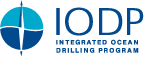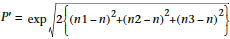IODP Proceedings    Volume contents     Searchdoi:10.2204/iodp.proc.314315316.222.2015

## Appendix

Below is the list of AMS parameters used in Table T1 and their mathematical expression.

 Km = bulk magnetic susceptibility; (|K1| + |K2| + |K3|)/3 SI.
 K1, K2, K3 = principal normed susceptibilities (K1  >  K2  >  K3),

where n1, n2 and n3 are their respective natural logarithms.

 L = K1/K2
 F = K2/K3
 P = K1/K3T = (2n2 – n1 – n3)/(n1 – n3)
 q = (K1 – K2)/{(K1 + K2)/2 – K3}
 K1 dec = declination of K1 in core coordinate system
 K1 inc = inclination of K1 in core coordinate system
 K2 dec = declination of K2 in core coordinate system
 K2 inc = inclination of K2 in core coordinate system
 K3 dec = declination of K3 in core coordinate system
 K3 inc = inclination of K3 in core coordinate system